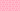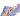PricingResources

# What is a risk-free interest rate?

Written by

Even the safest investments have an element of risk. Because of this, investors will often demand a rate of return that reflects inflationary expectations. That’s where the risk-free interest rate comes into play. Find out everything you need to know about the risk-free interest rate, including how to calculate it and what it means for businesses and investors. First off, what is a risk-free interest rate?

## Risk-free interest rate explained

The risk-free interest rate, also referred to as the risk-free rate of return, is a theoretical interest rate of an investment which carries zero risk. In actual terms, the risk-free interest rate is assumed to be equal to the interest rate paid on a three-month government Treasury bill, which is considered to be one of the safest investments that it’s possible to make.

Technically, the risk-free interest rate is purely theoretical, as all investments have some type of risk attached to them. Having said that, although it is possible for the government to default on its securities, the likelihood of this happening is enormously low. In fact, the level of risk is so low that it’s considered negligible. Hence, “risk-free”.

## Real risk-free interest rate vs. nominal risk-free interest rate

When we’re discussing risk-free interest rates, we’re essentially talking about two different things: the real risk-free interest rate and the nominal risk-free interest rate. Although it may sound complicated, the reasoning behind these concepts isn’t too difficult to grasp.

Essentially, the real risk-free interest rate refers to the rate of return required by investors on zero-risk financial instruments without inflation. Since this doesn’t exist, the real risk-free interest rate is a theoretical concept. However, while it cannot be observed in any meaningful way, studies have indicated that the real risk-free interest rate is equal to the economy’s long-run growth rate.

By contrast, the nominal risk-free interest rate is the observed return on a risk-free asset. You can work out the nominal risk-free interest rate using the real risk-free interest rate (however you decide to calculate it) and the inflation rate. The most important thing you need to understand about these two concepts is that their relationship is determined by the inflation rate.

## How to calculate the risk-free interest rate

There’s no consensus on how to calculate the risk-free interest rate, which means that there are a broad range of risk-free interest rate formulas that are purported to provide a direct measurement of the risk-free rate. Because of this, some analysts decide to look at so-called “proxies” for the risk-free interest rate. Short-dated government bonds, inter-bank lending rates, or AAA-rated corporate bonds from companies that are reportedly “too big to fail” are all good examples of potential proxies.

However, there are numerous issues with the proxy approach to calculating the risk-free interest rate. For example, government bonds can only really be risk-free if there’s no risk of default. However, while it’s extremely unlikely for these types of bonds to default, it does happen on occasion, which means that government bonds may not be a suitable proxy. Furthermore, there’s always a risk of the government “printing more money” to meet their obligations, leading to a loss of value.

Despite this, there are several risk-free interest rate formulas that can provide you with an effective way to calculate the risk-free interest rate. For example, you could simply subtract the current inflation rate from the Treasury bill’s yield over the duration of your investment. This calculation can be expressed using the following risk-free interest rate formula:

## What does the risk-free interest rate mean for businesses?

The risk-free interest rate has several important applications. First off, it plays an important role in a range of different financial calculations, including the Sharpe ratio and the Black-Scholes formula. In addition, businesses will need to pay attention to the risk-free interest rate, as rising risk-free rates could lead to higher required return rates from investors, thereby driving up the price of stock.

## We can help

GoCardless helps you automate payment collection, cutting down on the amount of admin your team needs to deal with when chasing invoices. Find out how GoCardless can help you with ad hoc payments or recurring payments.

Found this article interesting? Take a look at how to calculate Value at Risk (VaR)## Interested in automating the way you get paid? GoCardless can help

Contact sales

Sales

Contact Sales

+1(415) 523-2279

Support

help@gocardless.com

+1 (628) 241-0044